Refer to our Texas Go Math Grade 2 Answer Key Pdf to score good marks in the exams. Test yourself by practicing the problems from Texas Go Math Grade 2 Lesson 10.1 Answer Key Break Apart 3-Digit Addends.

Explore

Write the number. Draw a quick picture for the number. Then write the number in different ways.
____________ hundreds __________ tens ___________ ones
____________ + ____________ + ____________

____________ hundreds __________ tens ___________ ones
____________ + ____________ + ____________

FOR THE TEACHER • Have children write 258 on the blank In the left corner of the first box Have children draw a quick picture for this number and then complete the other two form for the number. Repeat the activity for 325.
258 = 200 hundreds 50 tens 8 ones
200 + 50 + 8

325 = 3 hundreds 2 tens 5 ones
300 + 20 + 5

Math Talk
Mathematical Processes

What number can be written as 400 + 20 + 9? Explain.
429

Explanation:
400+20+9 = 429
So, the number is 429.

Model and Draw

Break apart the addends into hundreds, tens, and ones. Add the hundreds, the tens, and the ones.
Then find the total sum.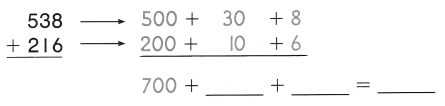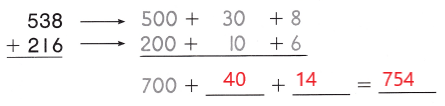Explanation:
The sum of 538 and 216 is 754.

Share and Show

Break apart the addends to find the sum.

Question 1.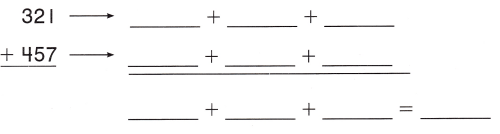Explanation:
The sum of 321 and 457 is 778.

Question 2.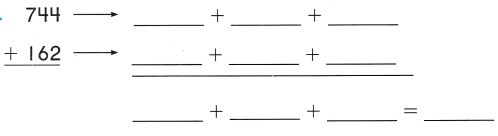Explanation:
The sum of 744 and 162 is 906.

Question 3.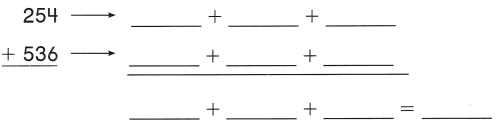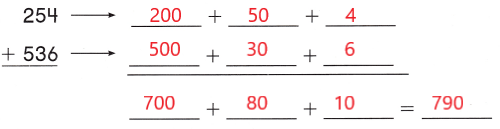Explanation:
The sum of 254 and 536 is 790.

Problem Solving

Break apart the addends to find the sum.

Question 4.Explanation:
The sum of 374 and 518 is 892.
Solve. Write or draw to explain.

Question 5.
H.O.T. Multi-Step Mr. Jones has 158 sheets of blue paper. 100 sheets of red paper, and 231 sheets of green paper. How many sheets of paper does he have?___________ sheets of paper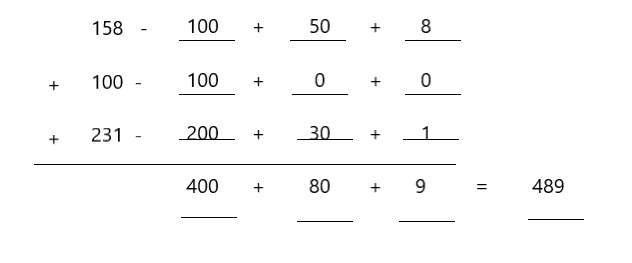Explanation:
The sum of 158, 100 and 231 is 489.

Question 6.
H.O.T. Wesley added in a different way.Use Wesley’s way to find the sum.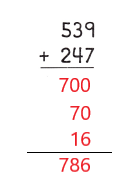Explanation:
The sum of 539 and 247 is 786.

Question 7.
Jeremy collected 329 acorns on Saturday and 263 acorns on Sunday. How many acorns did he collect?
(A) 599
(B) 589
(C) 592
(C) 592

Explanation:
Jeremy collected 329 acorns on Saturday
263 acorns on Sunday
329 + 263 = 592
So, they collected 592 acorns.

Question 8.
Analyze Daryl collects 112 stickers. Millie collects 293 stickers. How many stickers do they collect?
(A) 405
(B) 395
(C) 415
(A) 405

Explanation:
Daryl collects 112 stickers.
Millie collects 293 stickers.
112 + 293 = 405
So, they collected 405 stickers.

Question 9.
There are 452 cars on one side of the mall. There are 348 cars on the other side of the mal How many cars are there in all?
(A) 700
(B) 800
(C) 788
(B) 800

Explanation:
There are 452 cars on one side of the mall.
There are 348 cars on the other side of the mall
452 + 348 = 800
SO, there are 800 cars  there in all.

Question 10.
TEXAS Test Prep There are 321 children at Theo’s school. There are 419 children at Latasha’s school. How many children are at the two schools?
(A) 740
(B) 733
(C) 825
(A) 740

Explanation:
There are 321 children at Theo’s school.
There are 419 children at Latasha’s school.
321 + 419 = 740
There are 740 children at the two schools

TAKE HOME ACTIVITY • Write 347 + 215. Have your child break apart the numbers and then find the sum.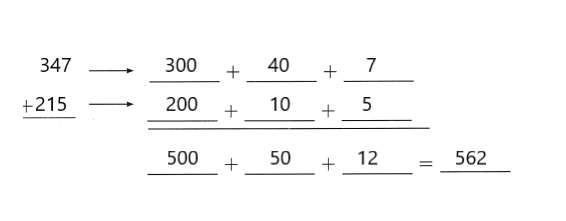Explanation:
The sum of 347 and 215 is 562.

### Texas Go Math Grade 2 Lesson 10.1 Homework and Practice Answer Key

Break apart the addends to find the sum.

Question 1.Explanation:
The sum of 246 and 321 is 567.

Question 2.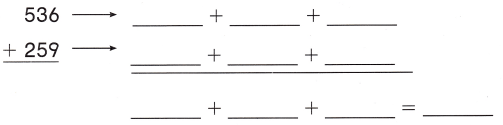Explanation:
The sum of 536 and 259 is 795.

Question 3.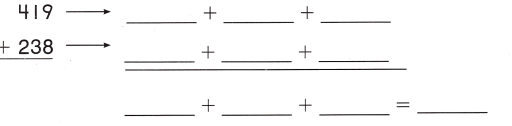Explanation:
The sum of 419 and 238 is 657.

Problem Solving

Solve. Write or draw to explain.

Question 4.
There are 108 carrots, 121 beans, and 165 tomatoes. How many vegetables are there?
___________ vegetables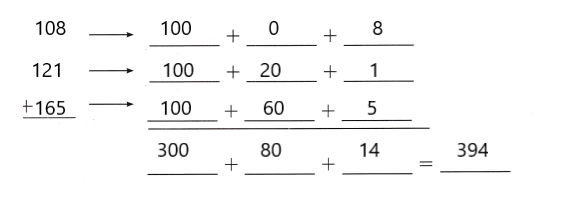Explanation:
The sum of 108, 121 and 165 is 394.

Lesson Check

Question 5.
There are 234 children at Marc’s day camp. There are 229 children at Lena’s day camp. How many children are at the two day camps?
(A) 463
(B) 453
(C) 535
(A) 463

Explanation:
Alan scored 254 points in a board game.
His friend Jess scored 272 points
234 + 229 is 463
So, there are 463 children at the two day camp.

Question 6.
Alan scored 254 points in a board game. His friend Jess scored 272 points. How many points did they score altogether?
(A) 426
(B) 536
(C) 526
(C) 526

Explanation:
Alan scored 254 points in a board game.
His friend Jess scored 272 points
254 + 272 = 526
So, they scored 526 altogether.

Question 7.
Max and Ruby are collecting acorns. Max collects 116 acorns. Ruby collects 145 acorns. How many acorns do they collect together?
(A) 251
(B) 261
(C) 361
(B) 261

Explanation:
Max and Ruby are collecting acorns.
Max collects 116 acorns.
Ruby collects 145 acorns
116 + 145 = 261
So, They collected 261 acorns in all.

Question 8.
Nolan and Sadie are picking up trash on their street. Nolan picks up 138 pieces of trash. Sadie picks up 165 pieces of trash, How many pieces of trash have they picked up?
(A) 293
(B) 313
(C) 303